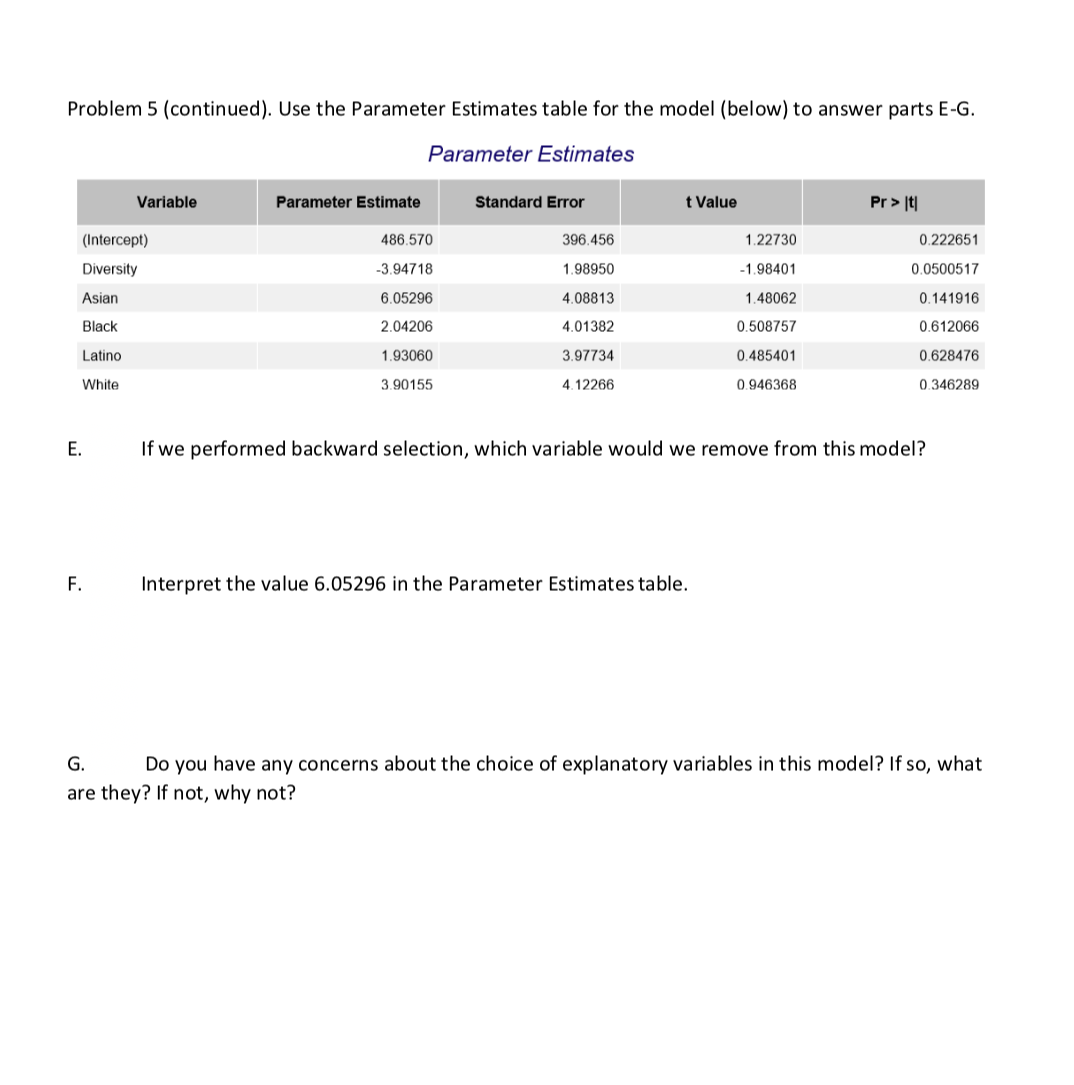# Problem 5 (continued). Use the Parameter Estimates table for the model (below) to answer parts E-G.Parameter EstimatesVariablet ValuePr > ItParameter EstimateStandard Error486.5703.947186.052962.042061.930603.90155396.4561.989504.088134.013823.977344.122660.2226510.05005170.1419160.6120660.6284760.3462891227301.984011.480620.5087570.4854010.946368(Intercept)DiversityAsianBlackLatinoWhiteE.If we performed backward selection, which variable would we remove from this model?Interpret the value 6.05296 in the Parameter Estimates table.F.Do you have any concerns about the choice of explanatory variables in this model? If so, whatare they? If not, why not?

Questionhelp_outlineImage TranscriptioncloseProblem 5 (continued). Use the Parameter Estimates table for the model (below) to answer parts E-G. Parameter Estimates Variable t Value Pr > It Parameter Estimate Standard Error 486.570 3.94718 6.05296 2.04206 1.93060 3.90155 396.456 1.98950 4.08813 4.01382 3.97734 4.12266 0.222651 0.0500517 0.141916 0.612066 0.628476 0.346289 122730 1.98401 1.48062 0.508757 0.485401 0.946368 (Intercept) Diversity Asian Black Latino White E. If we performed backward selection, which variable would we remove from this model? Interpret the value 6.05296 in the Parameter Estimates table. F. Do you have any concerns about the choice of explanatory variables in this model? If so, what are they? If not, why not? fullscreen
check_circle

Step 1

Part E:

The backward elimination (or backward selection, as you have given here) method of forming a regression model first constructs a model with all the available predictors, then eliminates or removes the least significant predictor from the model and re-constructs the model with the remaining predictors, continuing such elimination and re-construction of model till all the remaining predictors are significant.

Now, it is known that, the smaller the p-value, the more likely it is to be statistically significant. Using this property, a method to identify the least significant predictor is by identifying the predictor with the highest p-value.

In the given output, the p-values for the individual significance of the variables are given in the column “Pr > |t|”. The highest p-value is 0.628476, corresponding to the variable “Latino”.

Thus, in case of backward elimination, the variable Latino is to be removed.

Step 2

Part F:

The “Parameter Estimate” value 6.05296 is the slope of corresponding to the variable “Asian”. It has a positive sign, indicating that the relationship of the variable Asian to the response variable is positive, that is, increase in this predictor will be associated with increase in the response variable. It is not mentioned what is the response variable in the question. However, it would not affect the logic of the explanation.

Now, although it is not mentioned in your question, it is understood that the variable “Asian” is an indicator variable. As customary in this kind of a situation, it can be assumed that this variable takes the value 1 if an individual is an Asian and takes the value 0 if they are not an Asian. Thus, an increase in the value of the variable “Asian” from 0 to 1 implies a comparison of an Asian with a non-Asian.

In this multiple regression model, this can be interpreted as follows:

The value of the response variable is 6.05296 units more for an Asian than for a non-Asian.

Step 3

Part G:

Although the nature of the variables is not mentioned in the given question, a careful observation of the output does suggest that all the predictor variables used in the model are categorical, whereas the response variable is clearly continuous. (The output is clearly that of an ordinary multiple regression analysis with a continuous response).

The variables “Asian”, “Black”, “Latino” and “White” are evidently indicator variables denoting the ethnicity of a person, whereas “Diversity” appears to be another categorical variable, denoting diversity...

### Want to see the full answer?

See Solution

#### Want to see this answer and more?

Solutions are written by subject experts who are available 24/7. Questions are typically answered within 1 hour.*

See Solution
*Response times may vary by subject and question.
Tagged in

### Statistics